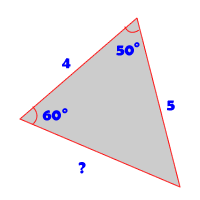# Solve for missing parts of a triangle

### Question:

If I'm given two side lengths and two angle measures of a triangle, how can I find the remaining side if the Pythagorean Theorem doesn't apply?$$\frac{a}{\sin{A}} = \frac{b}{\sin{B}} = \frac{c}{\sin{C}}$$
$$\frac{5}{\sin{60°}} = \frac{c}{\sin{50°}}$$ $$c = \frac{5*\sin{50°}}{\sin{60°}}$$ $$c = 4.42$$